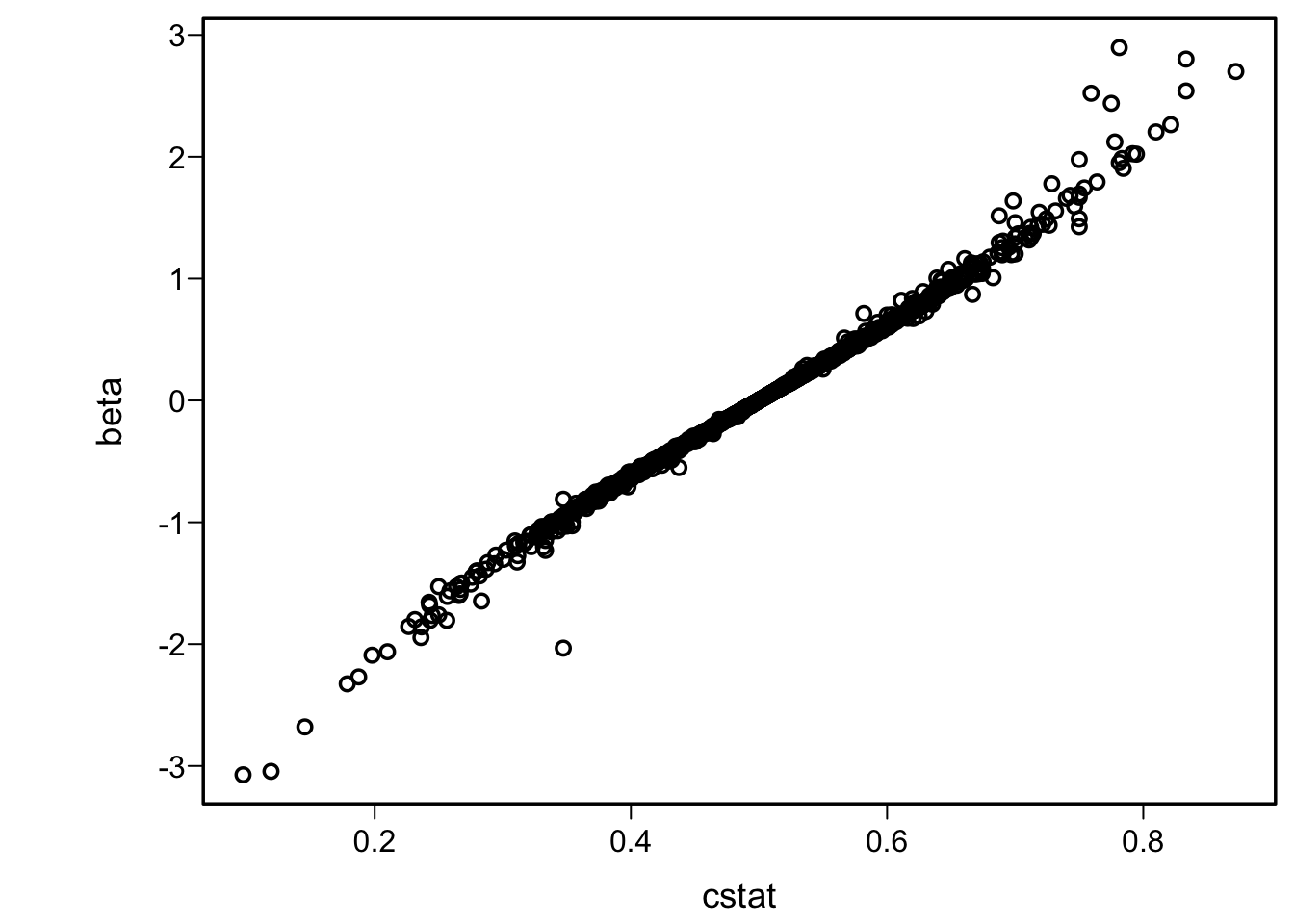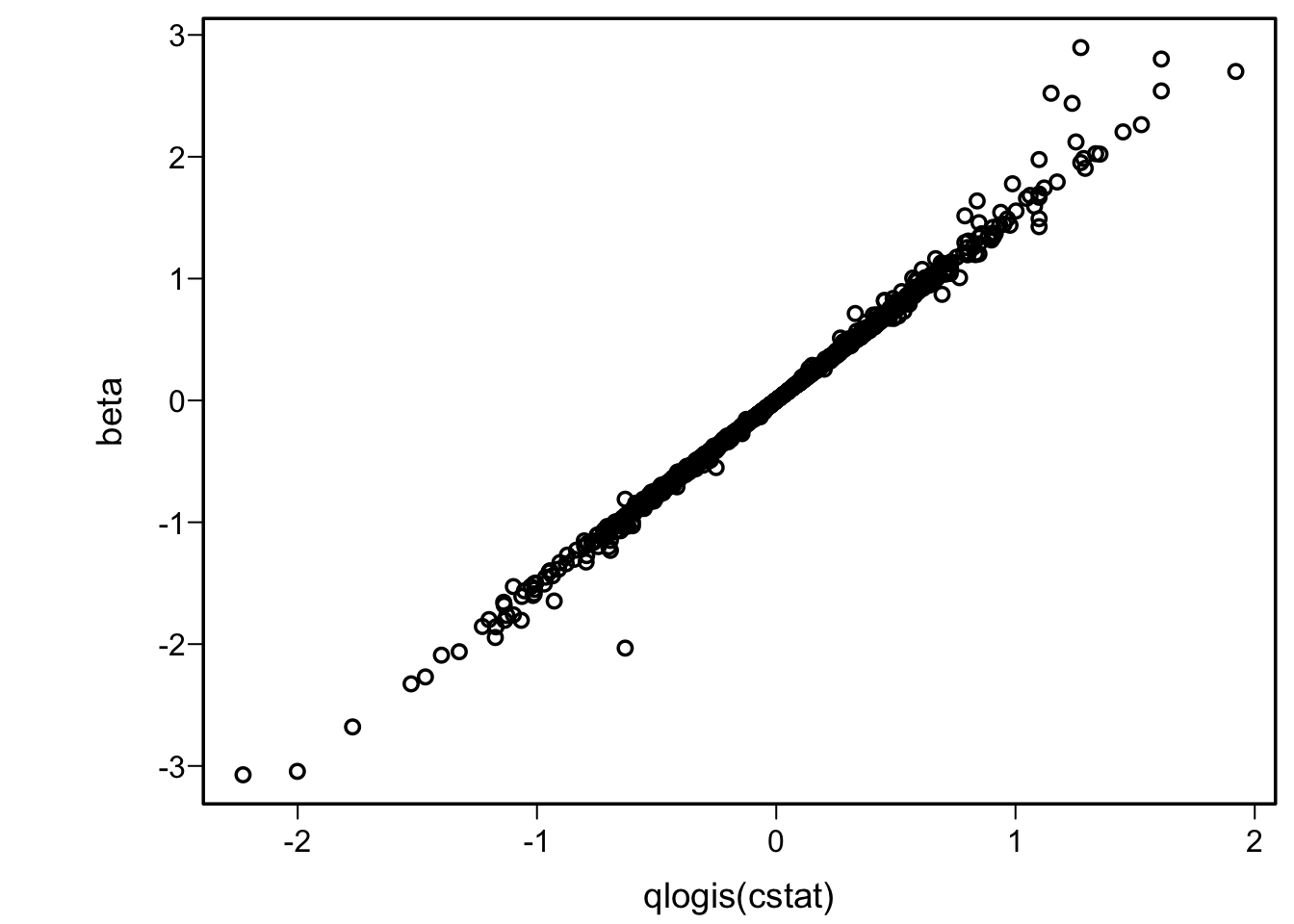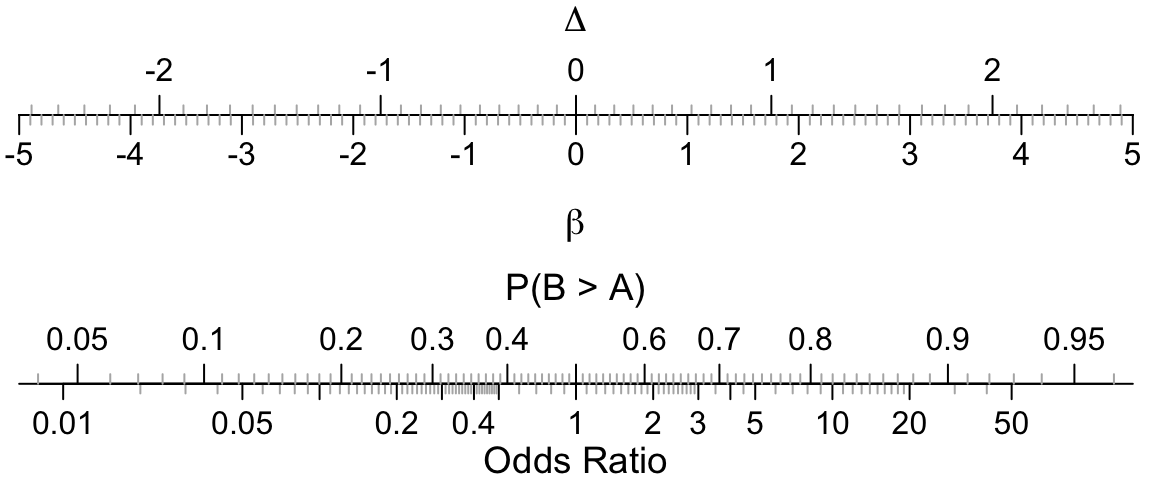# Violation of Proportional Odds is Not Fatal

2020
ordinal
accuracy-score
RCT
regression
hypothesis-testing
metrics
Many researchers worry about violations of the proportional hazards assumption when comparing treatments in a randomized study. Besides the fact that this frequently makes them turn to a much worse approach, the harm done by violations of the proportional odds assumption usually do not prevent the proportional odds model from providing a reasonable treatment effect assessment.
Author
Affiliation

Department of Biostatistics
Vanderbilt University School of Medicine

Published

September 20, 2020

Clearly, the dependence of the proportional odds model on the assumption of proportionality can be overstressed. Suppose that two different statisticians would cut the same three-point scale at different cut points. It is hard to see how anybody who could accept either dichotomy could object to the compromise answer produced by the proportional odds model. — Stephen Senn

# Background

The Wilcoxon Mann-Whitney (WMW) two-sample rank-sum test for comparing two groups on a continuous or ordinal outcome variable Y is a much liked statistical test, for good reason. It is robust to ‘outliers’ and is virtually as powerful as the two-sample t-test even if that test’s normality assumption holds. The k-sample generalization of the Wilcoxon test is the Kruskal-Wallis test, and a regression model generalization of both the Wilcoxon and Kruskal-Wallis tests is the proportional odds (PO) semiparametric logistic regression model for ordinal or continuous Y. The PO model has advantages over the WMW and Kruskal-Wallis tests in that it can handle covariate adjustment and allow for arbitrarily many ties in Y all the way to the case where Y is binary, at which point the PO model is the binary logistic model. The PO model also provides various useful estimates such as quantiles, means, and exceedance probabilities.

The WMW test involves the Mann-Whitney U statistic. U statistics were developed by Hoeffding in 1948. A U statistic is an average over all possible pairs (or triplets, quadruplets, etc.) of observations of a kernel. For WMW the kernel is a 0/1 indicator signifying whether a randomly chosen observation from treatment group B is larger than a randomly chosen observation in group A. So the Wilcoxon test tests whether values of Y in group B tend to be larger than those in group A, i.e., tests for stochastic ordering. The U statistic summary measures the degree to which Y values from B are separated from those in A, with a value of 0.5 indicating no tendency for values if B to be greater or less than those in A. This statistic is a concordance probability, also called a $$c$$-index. It is a measure of discrimination that is also equal to the area under a receiver operating characteristic curve. When treatments are denoted A and B, the $$c$$-index is the probability that a randomly chosen patient receiving treatment B has a value of Y that exceeds that of a randomly chosen patient receiving treatment A. It is a natural unitless parameter for quantifying treatment effect on an ordinal or continuous outcome.

For binary, ordinal, or continuous variables X and Y, rank correlation coefficients are excellent measures for quantifying the degree of association between X and Y. When X is binary such as a treatment indicator, rank correlation measures the separation of Y values that is apparently explained by treatment group X. The rank correlation coefficient that corresponds to the Wilcoxon test is Somers’ $$D_{yx}$$ where the order of $$yx$$ signifies that ties in x are ignored and ties in y are not. For comparing treatments A and B we do not draw a random pair of observations from the same treatment, but only consider observations in different treatment groups (drop ties in x). In the binary outcome situation, on the other hand, we use $$D_{xy}$$.

$$D_{yx}$$ is 0 if the $$c$$-index equals 0.5, i.e., there is no separation in Y values that can be explained by the treatment X. When c=0, meaning that all Y values for treatment A are larger than all Y values for B, $$D_{yx}=-1$$, and when c=1, meaning that all Y values for treatment B are larger than all values for treatment A (perfect separation in the opposite direction as when c=0), $$D_{yx}=1$$. There is a perfect correspondence between the MWM test, the concordance probability c, and Somers’ $$D_{yx}$$, i.e., you can perfectly compute the other two given any one of these indexes. $$D_{yx}$$ is also the difference between the probability of concordance of X and Y and the probability of discordance.

There is also a 1-1 relationship between the Wilcoxon statistic and Spearman’s $$\rho$$ rank correlation. $$\rho$$ is a different metric than $$D_{yx}$$ but it is possible to convert from one to the other for a given dataset.

For the normal case, i.e., when using a two-sample $$t$$-test or a linear model with a true treatment mean difference of $$\delta$$ and constant standard deviation of $$\sigma$$, the standard deviation of the difference between a randomly chosen response on treatment B and one from A is $$\sigma \sqrt{2}$$ since the variances sum. The concordance probability $$c$$ in this special case is $\begin{array}{lll} P(Y_{B} > Y_{A}) &=& P(Y_{B} - Y_{A} > 0) \\ &=& P(Y_{B} - Y_{A} - \delta > - \delta) \\ &=& P(Y_{B} - Y_{A} - \delta < \delta) \\ &=& P(\frac{Y_{B} - Y_{A} - \delta}{\sigma \sqrt{2}} < \frac{\delta}{\sigma \sqrt{2}}) \\ &=& P(Z < \frac{\delta}{\sigma \sqrt{2}}) \end{array}$ where $$Z$$ is a standard Normal(0,1) variable. If we let the standardized treatment difference (the difference in $$\sigma$$ units) be denoted by $$\Delta$$ (often called Cohen’s $$d$$), $$c = \Phi(\frac{\Delta}{\sqrt{2}})$$ where $$\Phi$$ denotes the standard normal cumulative distribution function.

# Relationship Between Log Odds Ratio and Rank Correlation

We use concordance probabilities or $$D_{yx}$$ without regard to the proportional odds (PO) assumption, and find them quite reasonable summaries of the degree to which Y increases when X increases. How then is the $$c$$-index related to the log odds ratio in the PO model whether or not the PO assumption is satisfied? There is no closed form solution for the maximum likelihood estimate $$\hat{\beta}$$ for treatment group in the PO model, but we can run a large number of simulations to describe the extent to which $$\hat{\beta}$$ tells us the same thing as $$c$$ or Somers’ rank correlation even when PO does not hold. Consider samples sizes of 10, 11, …, 100 and 1000 and take 20 random samples at each sample size. For each sample, take the treatment assignment x as a random sample of 0 and 1 each with probability 0.5, and take the Y values as a sample with replacement of the integers 1, …, n when the sample size is n. Sampling with replacement will create a variety of ties in the data. For each sample compute the concordance probability cstat and the maximum likelihood estimate for the x effect in the PO model. Because of the presence of sufficient randomness in the data generated (including large observed treatment effects in samples where there is none in the population), proportional odds will be apparently violated many times as judged by sample values.

Code
ns <- c(10:100, 1000)
d <- expand.grid(n = ns, m=1 : 20)
N <- nrow(d)
cstat <- beta <- numeric(N)
set.seed(5)
rn <- function(x) round(x, 3)
for(i in 1 : N) {
n <- d[i, 'n']
x <- sample(0 : 1, n, replace=TRUE)
y <- sample(1 : n, n, replace=TRUE)
cstat[i] <- somers2(y, x)['C']
# eps: stricter convergence criterion
b <- coef(orm(y ~ x, eps=0.000001, maxit=25))
beta[i] <- b[length(b)]
if(i == 3) {
cat('Sample with worst approximation\nbeta=',
rn(beta[i]), 'c=', rn(cstat[i]),
'\nbeta estimated from c:', rn(1.52 * qlogis(cstat[i])),
'\nc estimated from beta:', rn(plogis(beta[i] / 1.52)), '\n')
print(table(x, y))
saveRDS(data.frame(x, y), '/tmp/d.rds')
}
}
Sample with worst approximation
beta= -4.538 c= 0.031
beta estimated from c: -5.22
c estimated from beta: 0.048
y
x   1 2 3 4 6 9 10 11 12
0 0 0 0 0 1 0  1  1  1
1 2 1 1 3 0 1  0  0  0
Code
plot(cstat, beta)
plot(qlogis(cstat), beta)A very strong and linear relationship is found in the second graph above. I expected the second graph to be more linear, because the logit transformation of the concordance probability has an unlimited range just as the log odds ratio does. We can approximate $$\hat{\beta}$$ with a linear model in the logit of $$c$$:

Code
f <- ols(beta ~ qlogis(cstat))
f

Linear Regression Model

 ols(formula = beta ~ qlogis(cstat))

Model Likelihood
Ratio Test
Discrimination
Indexes
Obs 1840 LR χ2 10037.60 R2 0.996
σ 0.0396 d.f. 1 R2adj 0.996
d.f. 1838 Pr(>χ2) 0.0000 g 0.636

Residuals

        Min         1Q     Median         3Q        Max
-0.5527344 -0.0036478 -0.0004424  0.0022669  0.6742581

β S.E. t Pr(>|t|)
Intercept  0.0003  0.0009 0.37 0.7080
cstat  1.5179  0.0023 654.38 <0.0001
Code
r1 <- abs(beta - fitted(f))
r2 <- abs(cstat - plogis(beta / 1.52))
mae  <- rn(mean(r1))
cmae <- rn(mean(r2))
i    <- which.max(r1)
cat('<small>Maximum discrepency occurred in sample', i, '</small>\n')

Maximum discrepency occurred in sample 3

We see that $$\hat{\beta}$$ is very close to $$1.52 \times \mathrm{logit}(c)$$, or inverting the equation we get $$c = \frac{1}{1 + \exp(-\hat{\beta} / 1.52)}$$. The quality of the approximation is given by $$R^2$$=0.996 or by a mean absolute prediction error for $$\hat{\beta}$$ of 0.012. Predicting $$c$$ from $$\hat{\beta}$$ the mean absolute prediction error is 0.002.

Here is a graph that allows easy conversion from one metric to another. The axes are, in order from top to bottom, the standardized difference in means $$\Delta$$ if the response Y were to have a normal distribution (treatment regression coefficient in a linear model divided by the residual standard deviation), the log odds ratio $$\hat{\beta}$$ (treatment regression coefficient in a proportional odds model), the $$c$$-index (probability of treatment B having higher outcomes than treatment A), and odds ratio $$e^{\hat{\beta}}$$ (anti-log of $$\hat{\beta}$$).

Code
par(mar=c(3,.5,1,.5))
sdif   <- function(x, y) setdiff(round(x, 4), round(y, 4))
Delta  <- seq(-3, 3, by=1)
Delta2 <- sdif(seq(-3, 3, by=0.1), Delta)
conc   <- c(.01,.05,seq(.1,.9,by=.1),.95,.99)
conc2  <- sdif(seq(0.04, 0.96, by=0.01), conc)
or     <- c(0.01, 0.05, 0.1, 0.2, 0.3, 0.4, 0.5, 1, 2, 3, 4, 5, 10, 20, 50)
or2    <- sdif(c(seq(0.01, 0.5, by=0.01), seq(.5, 3, by=0.1), seq(3, 5, by=0.5),
6:20, 30, 40, 50), or)
lor    <- seq(-5, 5, by=1)
lor2   <- sdif(seq(-5, 5, by=0.1), lor)
f      <- function(conc) 1.52 * qlogis(conc)
g      <- function(z) f(pnorm(z / sqrt(2)))

plot.new()
par(usr=c(-5,5,-1.04,1.04))
par(mgp=c(1.5,.5,0))

axis(1, at=g(Delta), labels=as.character(Delta),
tcl=.5, mgp=c(-3,-1.7,0), line=-7)
axis(1, at=g(Delta2), tcl=.25, col.ticks=gray(.7), labels=FALSE, line=-7)
title(xlab=expression(Delta), line=-10)
axis(1, at=lor, as.character(lor),
tcl=-.5, line=-7)  # mgp=c(-3, 0.8, 0)
axis(1, at=lor2, tcl=-.25, col.ticks=gray(.7), labels=FALSE, line=-7)
title(xlab=expression(beta), line=-4.5)

axis(1, at=log(or),  labels=as.character(or))
axis(1, at=log(or2), tcl=-.25, col.ticks=gray(.7), labels=FALSE)
title(xlab='Odds Ratio')
axis(1, at=f(conc), labels=as.character(conc),
tcl=.5, mgp=c(-3,-1.7,0))
axis(1, at=f(conc2), tcl=.25, col.ticks=gray(.7), labels=FALSE)
title(xlab='P(B > A)', mgp=c(-3,-1.7,0))As discussed in Agresti and Kateri (see also Agresti and Tarantola), even with adjustment for covariates, if one compares a random patient on treatment B with a random patient on treatment A, with both patients having the same covariate values, the concordance probability is approximately $$\frac{1}{1 + \exp{(-\beta/\sqrt{2})}}$$ (this is exact for probit ordinal regression but not for logistic ordinal regression) whereas our better approximation replaces $$\sqrt{2} =$$ 1.41 with 1.52.

# Example

Consider an analysis of the relationship between cancer treatment (cisplatin vs. no cisplatin) and severity of nausea (6 levels) as analyzed in Peterson and Harrell 1990. First compute the exact concordance probability, which is a simple linear translation of the WMW statistic. Note that $$D_{xy}$$ in the output is really $$D_{yx}$$ since the order of arguments to the somers2 function was reversed.

Code
d0 <- data.frame(tx=0, y=c(rep(0, 43), rep(1, 39), rep(2, 13), rep(3, 22),
rep(4, 15), rep(5, 29)))
d1 <- data.frame(tx=1, y=c(rep(0, 7), rep(1, 7), rep(2, 3), rep(3, 12),
rep(4, 15), rep(5, 14)))
d <- rbind(d0, d1)
d$tx <- factor(d$tx, 0:1, c('No cisplatin', 'cisplatin'))
with(d, table(tx, y))
              y
tx              0  1  2  3  4  5
No cisplatin 43 39 13 22 15 29
cisplatin     7  7  3 12 15 14
Code
cindex <- with(d, somers2(y, as.numeric(tx) - 1))
cindex
          C         Dxy           n     Missing
0.6472478   0.2944956 219.0000000   0.0000000 
Code
cexact <- cindex['C']

Now use the rms package orm function to compute the overall cisplatin : no cisplatin log odds ratio for effect on severity of nausea.

Code
f <- orm(y ~ tx, data=d)
f

Logistic (Proportional Odds) Ordinal Regression Model

 orm(formula = y ~ tx, data = d)


Frequencies of Responses

  0  1  2  3  4  5
50 46 16 34 30 43

Model Likelihood
Ratio Test
Discrimination
Indexes
Rank Discrim.
Indexes
Obs 219 LR χ2 11.42 R2 0.052 ρ 0.229
Distinct Y 6 d.f. 1 R21,219 0.046
Y0.5 2 Pr(>χ2) 0.0007 R21,211.2 0.048
max |∂log L/∂β| 1×10-6 Score χ2 11.49 |Pr(Y ≥ median)-½| 0.059
Pr(>χ2) 0.0007
β S.E. Wald Z Pr(>|Z|)
y≥1   1.0189  0.1717 5.93 <0.0001
y≥2   0.0118  0.1546 0.08 0.9390
y≥3  -0.2996  0.1569 -1.91 0.0562
y≥4  -0.9868  0.1718 -5.75 <0.0001
y≥5  -1.7254  0.1994 -8.66 <0.0001
tx=cisplatin   0.9112  0.2714 3.36 0.0008
Code
b <- coef(f)['tx=cisplatin']
cest <- plogis(b / 1.52)

The concordance probability estimated from the average log odds ratio is 0.646 which is to be compared with the exact value of 0.647. The agreement is excellent despite non-PO, which is analyzed formally using a partial PO model here as well as in the Peterson and Harrell paper.

# Conclusion

When PO does not hold, the odds ratio from the proportional odds model represents a kind of average odds ratio, and there is an almost one-to-one relationship between the odds ratio (anti-log of $$\hat{\beta}$$) and the concordance probability $$c$$ (which is a simple translation of the Wilcoxon statistic). No model fits data perfectly, but as Stephen Senn stated in the quote that opened this article, the approximation offered by the PO model remains quite useful. And a unified PO model analysis is decidedly better than turning to inefficient and arbitrary analyses of dichotomized values of Y.

If you like the Wilcoxon test for comparing an ordinal response variable Y across treatments, or you like standard rank correlation measures for describing the strength of association between X and Y, you must like the PO model for summarizing treatment effects on ordinal (or continuous) Y. In a clinical trial we are interested in estimating the degree to which a treatment favorably redistributes patients across levels of Y in order to get a unified analysis of how a treatment improves patient outcomes. The PO model does that. And the natural parameter, concordance probability P(B > A), is a simple function of the PO model’s treatment odds ratio.

The place where a serious departure from the parallelism/PO assumption makes a large difference is in estimating treatment effects on individual outcome levels. For example, an overall odds ratio indicating that treatment benefits patients on an array of nonfatal outcomes may be in the opposite direction of how the treatment affects mortality. Though the overall average treatment effect estimated by assuming PO may rightfully claim a positive net clinical benefit of treatment, one can get a different picture when estimating the mortality effect ignoring all the other outcomes. This may be addressed using the partial proportional odds model of Peterson and Harrell, 1990 as implemented here and discussed in the COVID-19 context here.